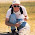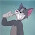## Saturday, September 19, 2015

### The difference between different methods for fitting the Mk model in R: It's all about the prior

There are multiple functions in R to fit the so-called Mk model for discrete character evolution. For instance, there is `ace` in the ape package, `fitDiscrete` in geiger, and now `fitMk` in my package, phytools - which has a lot of similarity to `ace`, but some important differences. This does not even consider the functions of phangorn and diversitree, which can also be used to fit this model.

A casual user may discovery, however, that although these methods all purport to be fitting the same model, parameter estimates and likelihoods often differ ever so slightly (but more than one would expect based on the limits of numerical precision) between methods.

What accounts for this, it turns out, is different implicit or explicit assumptions about the prior probability distribution at the global root of the tree. This is difficult to track down in documentation for any of these functions, although in `fitMk` the assumed prior (`pi`) is reported explicitly (and can be modified), and (as I show below) it is also possible to use `fitDiscrete` to fit the Mk model with any prior.

``````## load packages
library(phytools)
library(geiger)
``````

Next, let's simulate some data under the Mk model:

``````## simulate some data
tree<-pbtree(n=100,scale=1)
Q<-matrix(c(-2,1,1,1,-2,1,1,1,-2),3,3)
rownames(Q)<-colnames(Q)<-letters[1:3]
Q
``````
``````##    a  b  c
## a -2  1  1
## b  1 -2  1
## c  1  1 -2
``````
``````x<-sim.history(tree,Q,anc="a")\$states
``````
``````## Done simulation(s).
``````
``````x
``````
``````##  t53  t54  t89  t90   t9  t10   t6  t11  t24  t25  t12  t44  t62  t67  t74
##  "a"  "a"  "c"  "c"  "a"  "a"  "b"  "b"  "a"  "a"  "a"  "a"  "a"  "b"  "a"
##  t75  t65  t66  t45  t81  t82  t69  t35  t36  t70  t71   t1   t5  t17  t23
##  "a"  "c"  "c"  "a"  "a"  "c"  "a"  "a"  "a"  "b"  "c"  "c"  "a"  "a"  "a"
##  t91  t92  t55  t56  t46  t47  t50  t51  t22  t78  t85  t86  t61  t63  t64
##  "c"  "b"  "c"  "c"  "c"  "c"  "c"  "c"  "c"  "c"  "c"  "c"  "c"  "c"  "c"
##  t18  t95  t96  t13  t42  t43  t93  t94  t99 t100  t15   t4  t40  t41   t2
##  "c"  "b"  "b"  "c"  "c"  "c"  "c"  "c"  "a"  "a"  "b"  "c"  "b"  "b"  "b"
##   t3  t76  t77  t39  t38  t30  t27  t31  t83  t84  t57  t58  t37  t14  t19
##  "b"  "b"  "a"  "a"  "a"  "b"  "a"  "a"  "a"  "a"  "a"  "a"  "c"  "a"  "a"
##  t20   t7  t79  t80  t21   t8  t68  t87  t88  t52  t72  t73  t33  t34  t16
##  "a"  "c"  "b"  "b"  "b"  "a"  "a"  "a"  "a"  "a"  "b"  "b"  "b"  "b"  "b"
##  t26  t28  t29  t48  t49  t97  t98  t32  t59  t60
##  "b"  "c"  "a"  "a"  "a"  "b"  "b"  "b"  "a"  "b"
``````

Now, we can fit each model under the generating model (`model="ER"`):

``````## fit models
fit.phytools<-fitMk(tree,x,model="ER",pi="equal") ## flat root
fit.phytools
``````
``````## Object of class "fitMk".
##
## Fitted (or set) value of Q:
##           a         b         c
## a -1.773072  0.886536  0.886536
## b  0.886536 -1.773072  0.886536
## c  0.886536  0.886536 -1.773072
##
## Fitted (or set) value of pi:
##         a         b         c
## 0.3333333 0.3333333 0.3333333
##
## Log-likelihood: -86.449618
``````
``````fit.ape<-ace(x,tree,type="discrete",model="ER")
fit.ape
``````
``````##
##     Ancestral Character Estimation
##
## Call: ace(x = x, phy = tree, type = "discrete", model = "ER")
##
##     Log-likelihood: -85.35101
##
## Rate index matrix:
##   a b c
## a . 1 1
## b 1 . 1
## c 1 1 .
##
## Parameter estimates:
##  rate index estimate std-err
##           1   0.8865  0.1524
##
## Scaled likelihoods at the root (type '...\$lik.anc' to get them for all nodes):
##         a         b         c
## 0.4114971 0.3071304 0.2813725
``````
``````fit.geiger<-fitDiscrete(tree,x,model="ER")
fit.geiger
``````
``````## GEIGER-fitted comparative model of discrete data
##  fitted Q matrix:
##                a          b          c
##     a -1.7668479  0.8834239  0.8834239
##     b  0.8834239 -1.7668479  0.8834239
##     c  0.8834239  0.8834239 -1.7668479
##
##  model summary:
##  log-likelihood = -86.421424
##  AIC = 174.842847
##  AICc = 174.883663
##  free parameters = 1
##
## Convergence diagnostics:
##  optimization iterations = 100
##  failed iterations = 0
##  frequency of best fit = 1.00
##
##  object summary:
##  'lik' -- likelihood function
##  'bnd' -- bounds for likelihood search
##  'res' -- optimization iteration summary
##  'opt' -- maximum likelihood parameter estimates
``````

We can see that each is slightly different - either in the fitted model, or the likelihood, or both!

To show that this is due to different priors on the root node, we can change the prior using the object created by `fitDiscrete`. This is not super-straightforward, but kind of neat in the end. (There may be some way to do this within a call of `fitDiscrete`, but I was not able to do that.)

``````## the likelihood function
lik<-fit.geiger\$lik
## optimize it using a flat/"equal" prior
fit.flat<-optimize(lik,c(1e-8,1000),root=ROOT.FLAT,maximum=TRUE)
fit.flat
``````
``````## \$maximum
##  0.8865379
##
## \$objective
##  -86.44962
``````

If we compare this rate parameter to `fitMk` or `ace`, or the likelihood to `fitMk`, we should see that it is equal. Cool.

1.Great post Liam,

Another option out their is the way that diversitree handles this by default. Where the likelihoods at the root are weighted in proportion to how well they explain the observed tip data. Rich does a nice job of explaining the approach in the first appendix of Fitzjohn, Maddison and Otto 2009. Also in diversitree you can choose just to return the multiple probabilites calculated at the root rather than summing over them. This can be nice at least for exploration so you can see how or to what extent the choice of root prior is going to effect your result

cheers

2.This comment has been removed by the author.

3.The replica watches uk
has your name from the digital dial. However, this does not necessarily mean that the watch is also operated digitally. You

can combine this type of watch with puristic outfits in black or white. So the special look of the replica-rolexcomes over well.

4.This is one of the best posts i have seen inbuy replica watches a long time. thanks for posting it. while there are different grades of replicas,( some better than others) it is best to read the reviews from the owners on these boards to find out replica Omega watches UKthe real quality of a watch you are wanting to purchase. never listen to a seller just arbitrarily saying his product is grade 1 or AAA. he only wants your money.

5.Why is the likelihood returned by ace (-85.35101) not the same as the likelihood returned by the geiger likelihood function (-86.44962), when evaluated at the same rate value (0.8865379)? The examples in the help page for fitDiscrete give a clue: one can get the same likelihood as fitMk (-86.44962) like this:

fit(0.8865379, root="given", root.p=c(1/3,3))

or one can get the same likelihood as ace (-85.35101) like this, using un-normalized root probabilities (1,1,1 instead of .33,.33,.33):

fit(0.8865379, root="given", root.p=c(1,3))

So it looks like the likelihood output by ace has an extra term log(3) = 1.098612 = -85.35101 - (- 86.44962). That's log(3) for 3 states. Weird. It's the same constant term for all choices of rate parameters, so the rates estimated by ace are correct. Any likelihood ratio would still be correct, too. But still, I find this strange.

Thanks Liam for your post, and thanks Luke for the help in the manual page of fitDiscrete!

Note: due to the very large amount of spam, all comments are now automatically submitted for moderation.